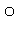## Optimization flags

Each internal coordinate is followed by an flag, to indicate the action to be taken.

 Flag Action 1 Optimization Flags 0 Do not optimize the coordinate. -1 Reaction coordinate, or grid index. T Monitor turning points in DRC 2 The same as "1", but used in deciding which atoms to print (see MINI)

Consider the data set for formaldehyde:

``` SYMMETRY
Formaldehyde

O  0.0 0    0.0 0    0.0 0
C  1.2 1    0.0 0    0.0 0  1  0  0
H  1.1 1  120.0 1    0.0 0  2  1  0
H  1.1 0  120.0 0  180.0 0  2  1  3

3  1    4
3  2    4 ```
The optimization flags for the first atom, oxygen, are all zero, because the system is in internal coordinates and translating the molecule would not alter its energy at all.

For atom 2, carbon, the first coordinate, the C=O bond length, 1.2Å, is flagged for optimization, the other two coordinates are not flagged for optimization, because there is no angle or dihedral.

Atom 3, hydrogen, has both the C-H bond length, 1.1Å, and the H-C=O angle, 120 degrees, flagged for optimization.

Atom 4, also a hydrogen, has no coordinates marked for optimization, because the C-H and H-C=O parameters are symmetry-defined to be the same as those of atom 3, and the dihedral is exactly 180 degrees.

Only one reaction coordinate is allowed, but this can be made more versatile by the use of SYMMETRY. Two methods of specifying the points to be used on the reaction coordinate are allowed.

#### Using explicit points

If the user wants to explicitly specify each point, then the values of the reaction coordinate should follow immediately after the geometry and any symmetry data. No terminator is required, and free-format-type input is acceptable.   For each point calculated, this option produces an output that is similar to the normal MOPAC output for a geometry optimization.

#### Using a step-size

If the points to be used form a regular sequence, then the preferred method of specifying them is by use of the two keywords POINT=n and STEP=n.nn. For example, to rotate a torsion angle through 360in steps of 10, the keywords POINT=36 STEP=10 would be used.  For each point calculated, this option produces an output that consists of the values of the variable and the heat of formation, and the geometry at that point, i.e., the output is much smaller than that of a normal geometry optimization.

#### Two-dimensional grid

If two "reaction coordinates" are used, then MOPAC assumes that the two-dimensional space in the region of the supplied geometry is to be mapped. The two dimensions to be mapped are in the plane defined by the "-1" labels. Step sizes in the two directions must be supplied using STEP1 and STEP2 on the keyword line.

Using internal coordinates, the first atom has three unoptimizable coordinates, the second atom two, (the bond-length can be optimized) and the third atom has one unoptimizable coordinate. None of these six unoptimizable coordinates at the start of the geometry should be marked for optimization. If any are so marked, a warning is given, but the calculation will continue.

In Cartesian coordinates all parameters can be optimized.

In IRC/DRC calculations, the flag "T" specifies that turning points for the coordinate are to be printed, see Dynamic and Intrinsic Reaction Coordinates for more detail.